We use cookies to give you the best possible experience while using our website.

Got it!

### Chapter One - Introduction to the Thai Tones

##### Thai Mid Tone Sound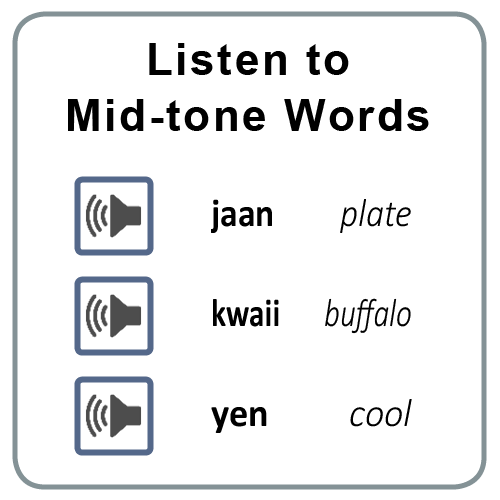This introduction to the Thai language can help you to understand about the Thai consonant classes, long and short vowels, and the five Thai tones.

##### Thai Low Tone Sound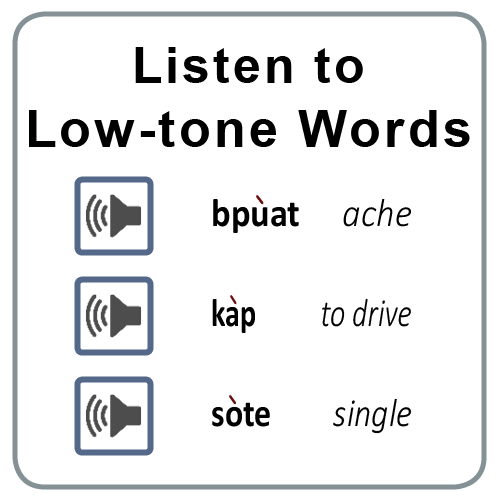This introduction to the Thai language can help you to understand about the Thai consonant classes, long and short vowels, and the five Thai tones.

##### Thai Falling Tone Sound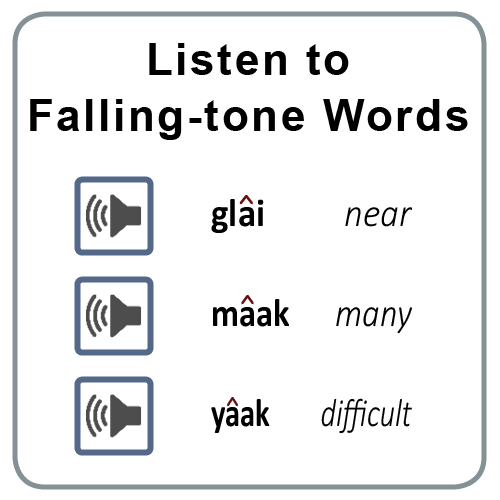This introduction to the Thai language can help you to understand about the Thai consonant classes, long and short vowels, and the five Thai tones.

##### Thai High Tone Sound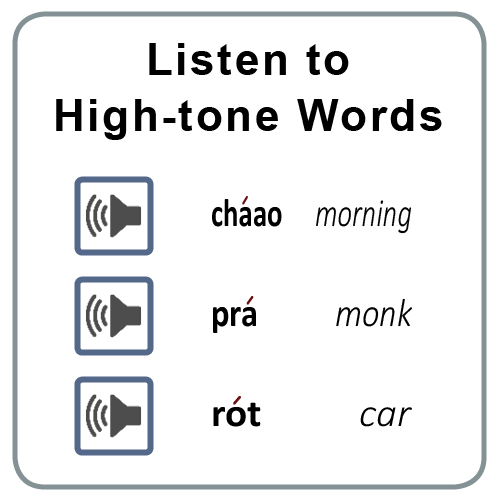This introduction to the Thai language can help you to understand about the Thai consonant classes, long and short vowels, and the five Thai tones.

##### Thai Rising Tone Sound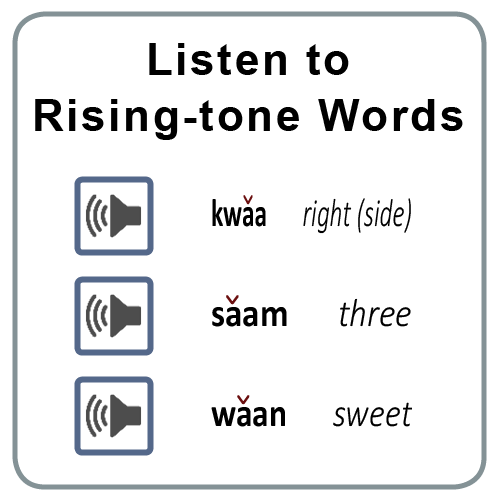This introduction to the Thai language can help you to understand about the Thai consonant classes, long and short vowels, and the five Thai tones.

##### Hearing the Five Tones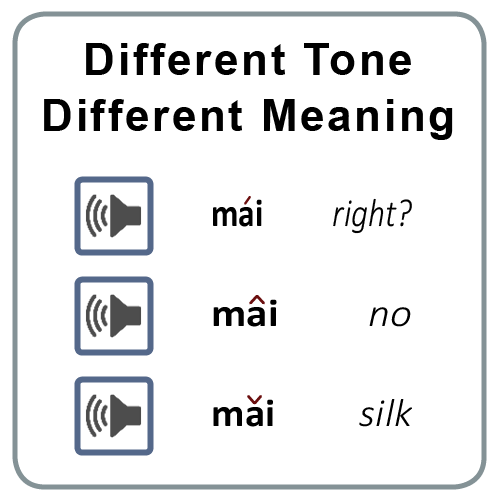This introduction to the Thai language can help you to understand about the Thai consonant classes, long and short vowels, and the five Thai tones.

##### The Five Tones TestThis introduction to the Thai language can help you to understand about the Thai consonant classes, long and short vowels, and the five Thai tones.

### Chapter Two - The 44 Thai Consonants

##### The Middle Class ConsonantsIn this lesson you will start learning the Thai alphabet. Though not a true alphabet, the Thai writing system consists of 44 consonants, 32 vowels, and several punctuation marks.

##### The High Class ConsonantsIn this lesson you will start learning the Thai alphabet. Though not a true alphabet, the Thai writing system consists of 44 consonants, 32 vowels, and several punctuation marks.

##### The Low Class Consonants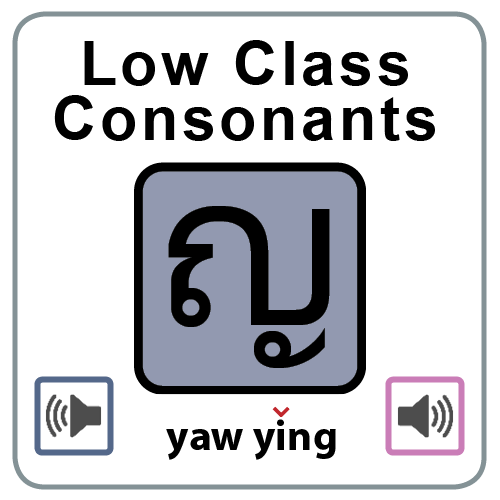In this lesson you will start learning the Thai alphabet. Though not a true alphabet, the Thai writing system consists of 44 consonants, 32 vowels, and several punctuation marks.

##### The Consonant Names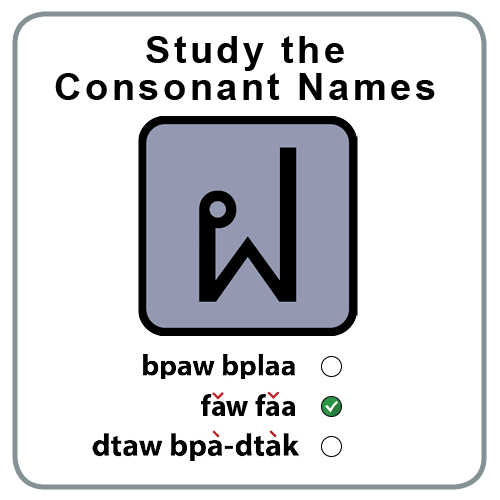In this lesson you will start learning the Thai alphabet. Though not a true alphabet, the Thai writing system consists of 44 consonants, 32 vowels, and several punctuation marks.

##### Consonant Names Test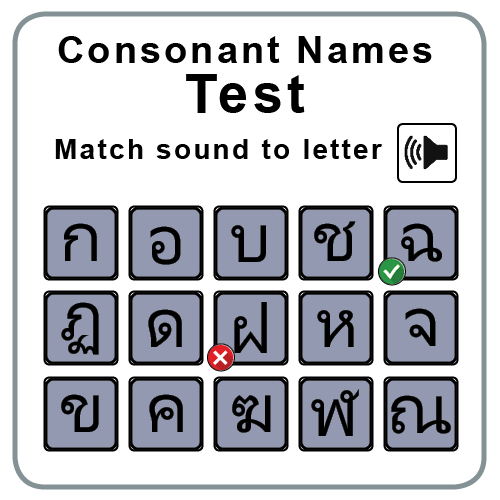In this lesson you will start learning the Thai alphabet. Though not a true alphabet, the Thai writing system consists of 44 consonants, 32 vowels, and several punctuation marks.

##### The Consonant Classes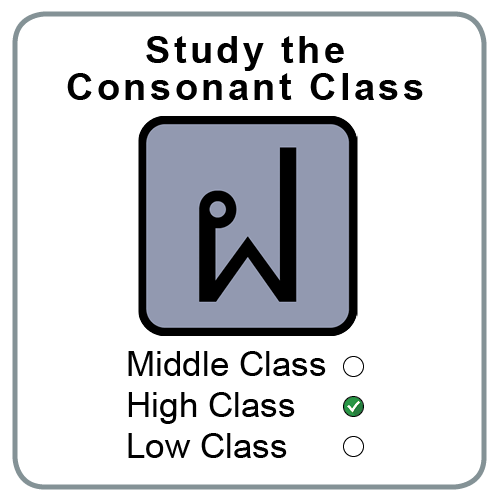In this lesson you will start learning the Thai alphabet. Though not a true alphabet, the Thai writing system consists of 44 consonants, 32 vowels, and several punctuation marks.

##### Middle Class Consonants Test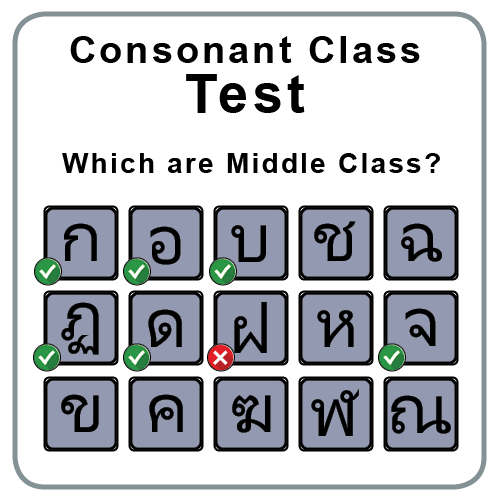In this lesson you will start learning the Thai alphabet. Though not a true alphabet, the Thai writing system consists of 44 consonants, 32 vowels, and several punctuation marks.

##### High Class Consonants Test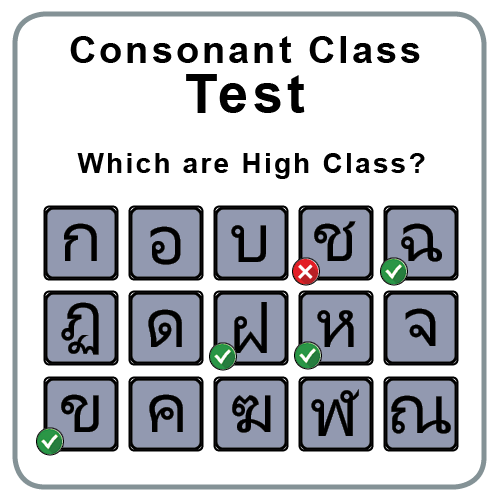In this lesson you will start learning the Thai alphabet. Though not a true alphabet, the Thai writing system consists of 44 consonants, 32 vowels, and several punctuation marks.

##### Low Class Consonants Test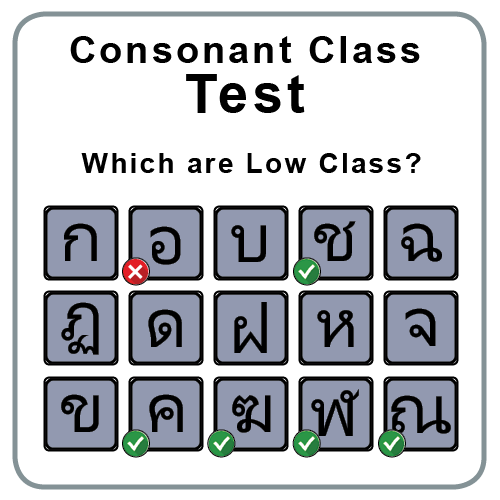In this lesson you will start learning the Thai alphabet. Though not a true alphabet, the Thai writing system consists of 44 consonants, 32 vowels, and several punctuation marks.

##### Listen and Type the Thai Consonants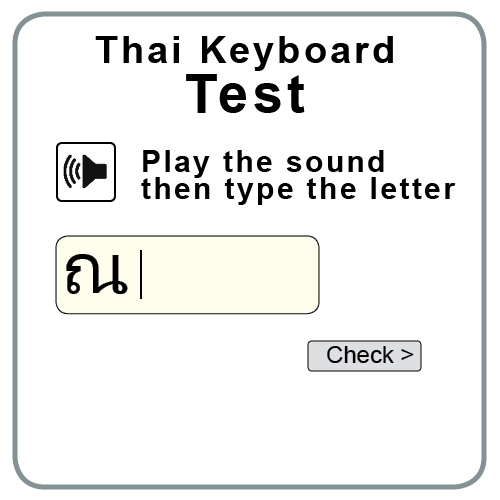In this lesson you will start learning the Thai alphabet. Though not a true alphabet, the Thai writing system consists of 44 consonants, 32 vowels, and several punctuation marks.

##### Listen and Write the Thai Consonants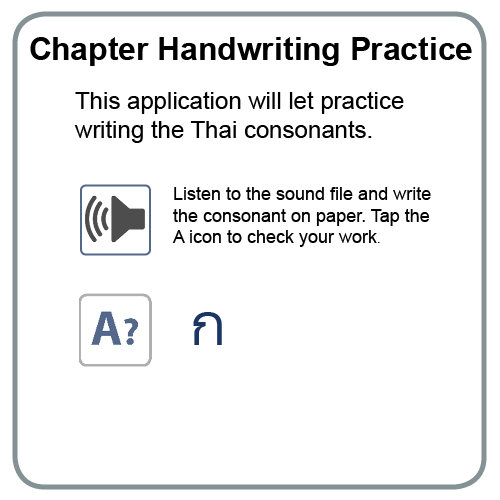In this lesson you will start learning the Thai alphabet. Though not a true alphabet, the Thai writing system consists of 44 consonants, 32 vowels, and several punctuation marks.

### Chapter Three - The 32 Thai Vowels

##### 12 Short Vowel Sounds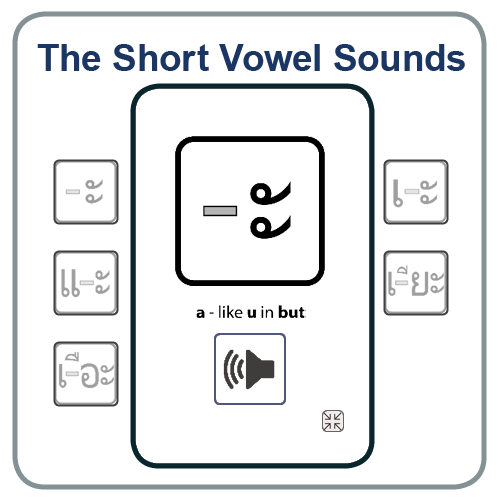In this lesson we will introduce the Thai vowels, and help you to understand the difference between the long, short, and diphthong vowel sounds of the Thai language, and how they effect the tone rules.

##### 12 Long Vowel Sounds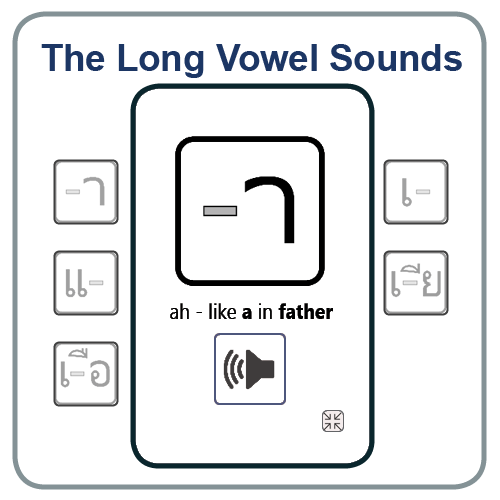In this lesson we will introduce the Thai vowels, and help you to understand the difference between the long, short, and diphthong vowel sounds of the Thai language, and how they effect the tone rules.

##### Eight Special Vowel Symbols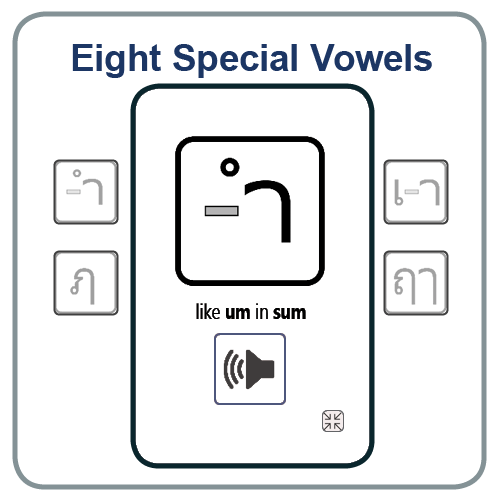In this lesson we will introduce the Thai vowels, and help you to understand the difference between the long, short, and diphthong vowel sounds of the Thai language, and how they effect the tone rules.

##### Compare Long & Short VowelsIn this lesson we will introduce the Thai vowels, and help you to understand the difference between the long, short, and diphthong vowel sounds of the Thai language, and how they effect the tone rules.

##### Long & Short Vowel Practice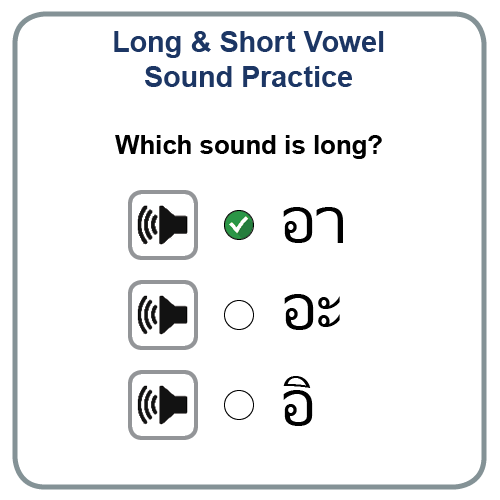In this lesson we will introduce the Thai vowels, and help you to understand the difference between the long, short, and diphthong vowel sounds of the Thai language, and how they effect the tone rules.

##### Practice The Thai Vowels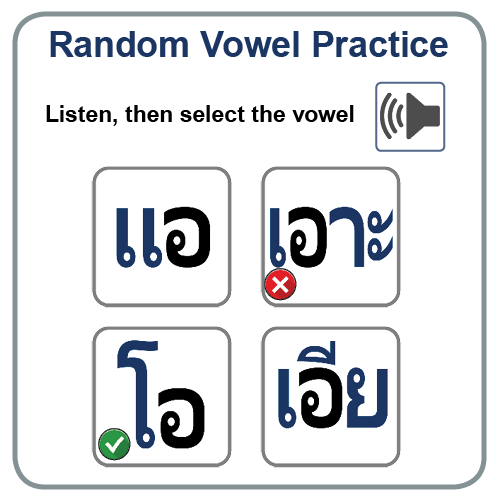In this lesson we will introduce the Thai vowels, and help you to understand the difference between the long, short, and diphthong vowel sounds of the Thai language, and how they effect the tone rules.

##### Identify the 14 Thai Short Vowels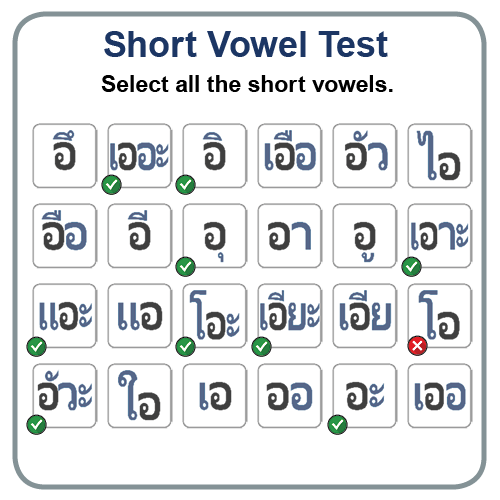In this lesson we will introduce the Thai vowels, and help you to understand the difference between the long, short, and diphthong vowel sounds of the Thai language, and how they effect the tone rules.

##### Identify the 18 Thai Long Vowels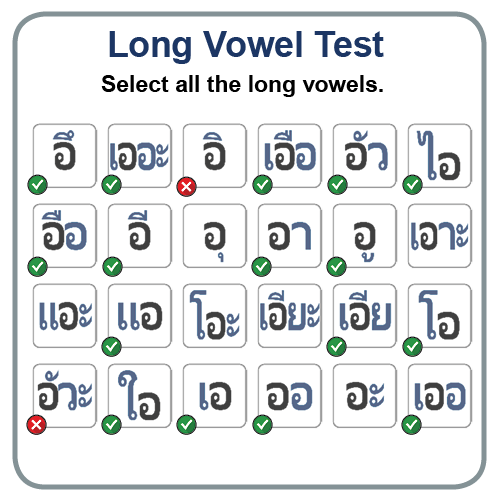In this lesson we will introduce the Thai vowels, and help you to understand the difference between the long, short, and diphthong vowel sounds of the Thai language, and how they effect the tone rules.

##### Listen and Type the Thai Vowels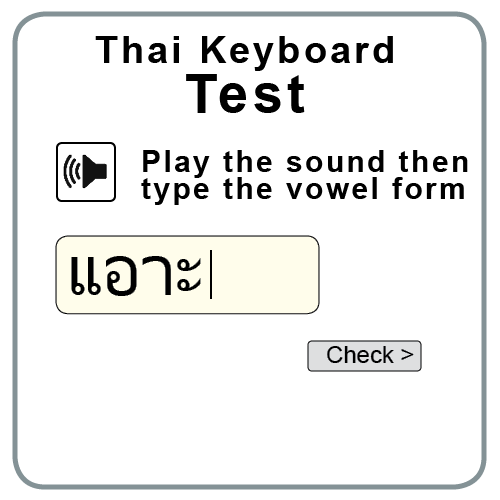In this lesson we will introduce the Thai vowels, and help you to understand the difference between the long, short, and diphthong vowel sounds of the Thai language, and how they effect the tone rules.

##### Listen and Write the Thai Vowels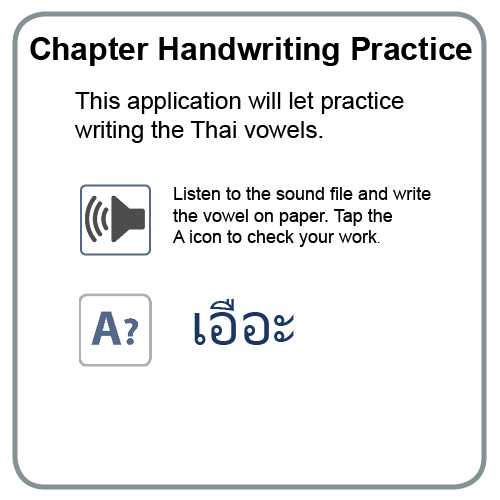In this lesson we will introduce the Thai vowels, and help you to understand the difference between the long, short, and diphthong vowel sounds of the Thai language, and how they effect the tone rules.

##### Complex Vowel Sounds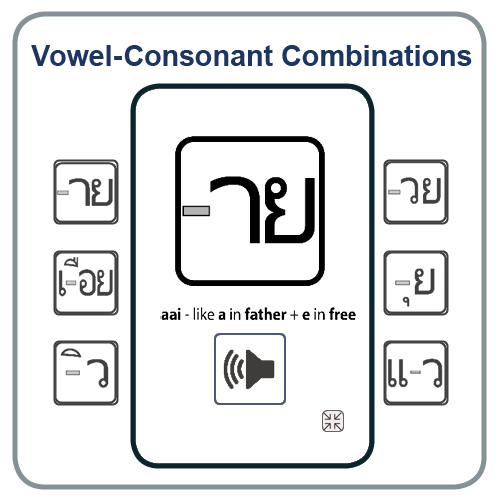In this lesson we will introduce the Thai vowels, and help you to understand the difference between the long, short, and diphthong vowel sounds of the Thai language, and how they effect the tone rules.

### Chapter Four - Spelling Thai Sounds

##### Consonant with a Vowel Sound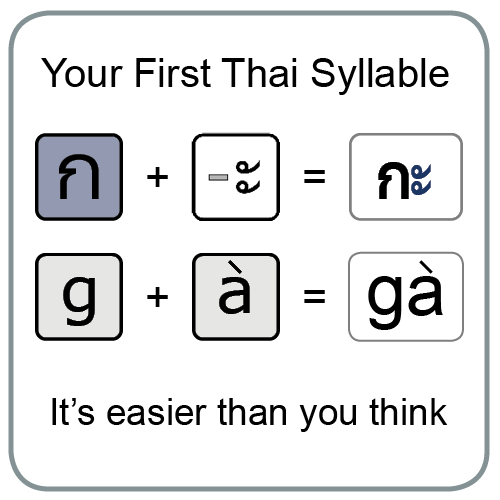In this lesson you will learn to spell out the sounds that make up Thai words. You've learned the consonants and vowels, so now let get down to the basics of how Thai syllables are formed.

##### The Thai Final Consonants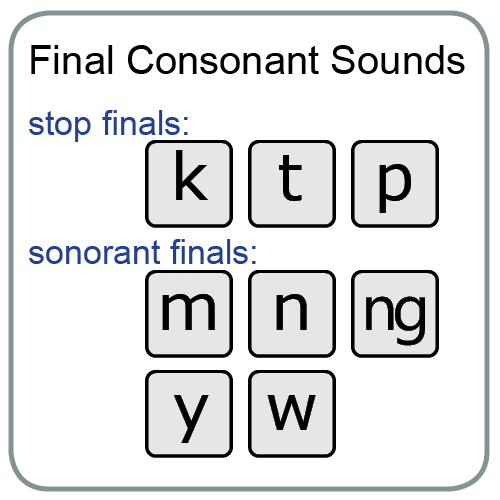In this lesson you will learn to spell out the sounds that make up Thai words. You've learned the consonants and vowels, so now let get down to the basics of how Thai syllables are formed.

##### Consonant, Vowel, Consonant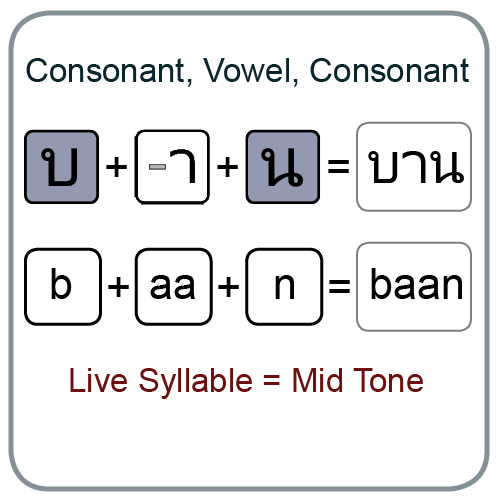In this lesson you will learn to spell out the sounds that make up Thai words. You've learned the consonants and vowels, so now let get down to the basics of how Thai syllables are formed.

##### Seven Vowels That Change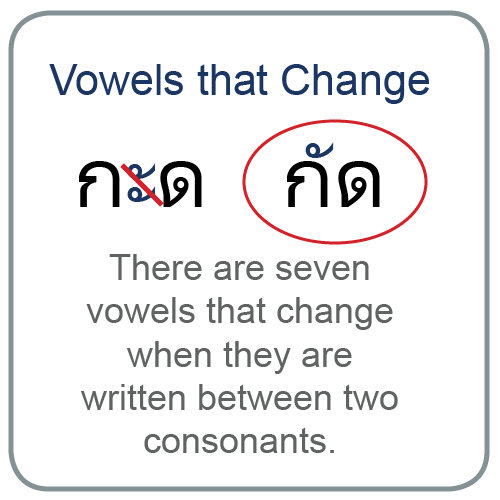In this lesson you will learn to spell out the sounds that make up Thai words. You've learned the consonants and vowels, so now let get down to the basics of how Thai syllables are formed.

##### Listen and Read Thai Syllables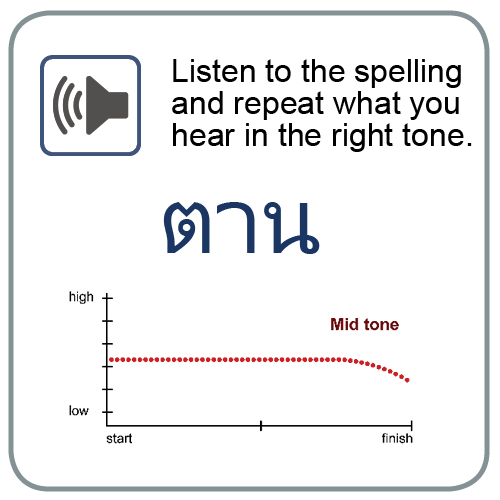In this lesson you will learn to spell out the sounds that make up Thai words. You've learned the consonants and vowels, so now let get down to the basics of how Thai syllables are formed.

##### Listen and Type Thai Syllables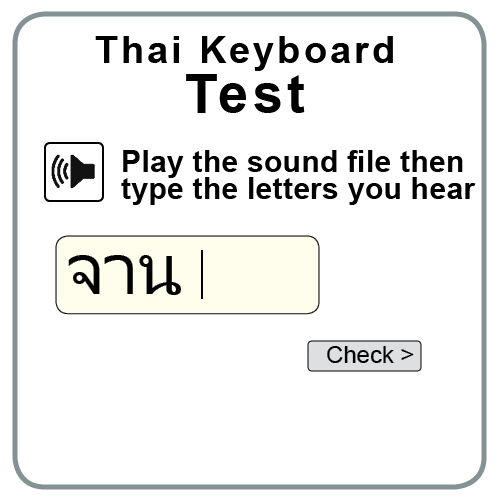In this lesson you will learn to spell out the sounds that make up Thai words. You've learned the consonants and vowels, so now let get down to the basics of how Thai syllables are formed.

##### Listen and Write Thai Syllables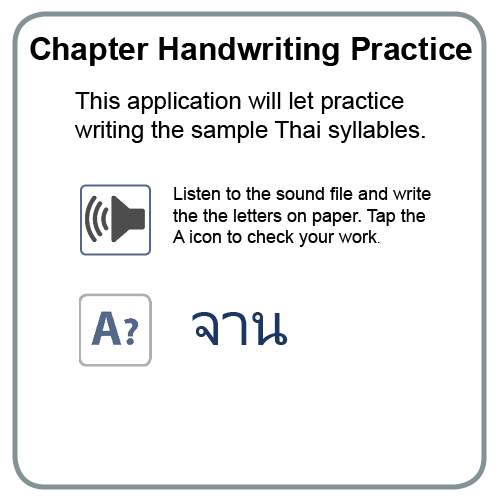In this lesson you will learn to spell out the sounds that make up Thai words. You've learned the consonants and vowels, so now let get down to the basics of how Thai syllables are formed.

### Chapter Five - Learning the Thai Tone Rules

##### Live and Dead Syllable Types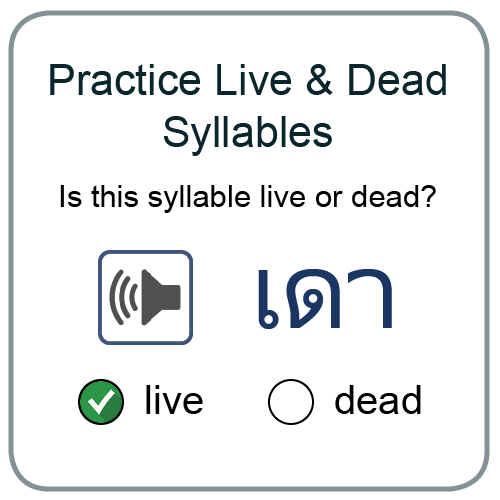In this chapter you will learn to put everything that you have learned about the Thai consonants together with vowels and the final consonant sounds to learn the tone rules, then learn how these rules are modified using one of the four Thai tone marks.

##### Practice Middle Class Syllables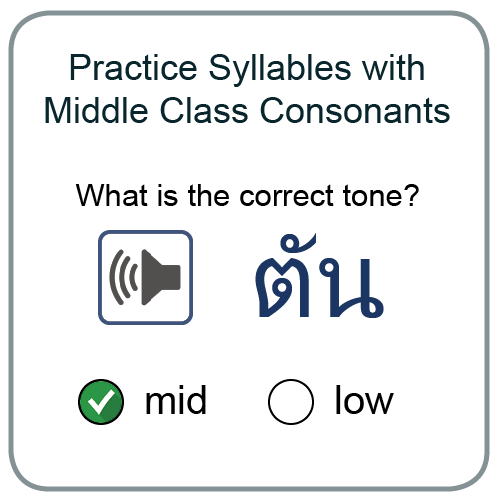In this chapter you will learn to put everything that you have learned about the Thai consonants together with vowels and the final consonant sounds to learn the tone rules, then learn how these rules are modified using one of the four Thai tone marks.

##### Tone Rules for Middle Class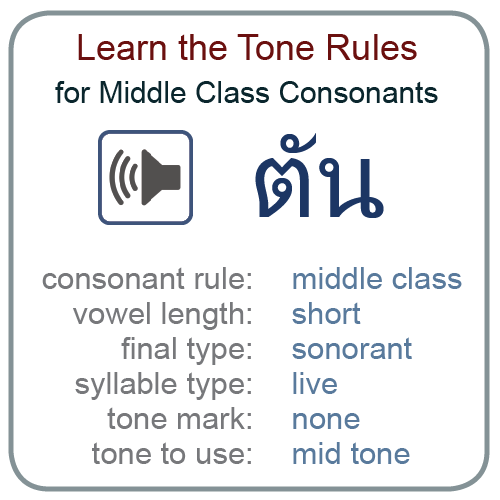In this chapter you will learn to put everything that you have learned about the Thai consonants together with vowels and the final consonant sounds to learn the tone rules, then learn how these rules are modified using one of the four Thai tone marks.

##### Practice High Class Syllables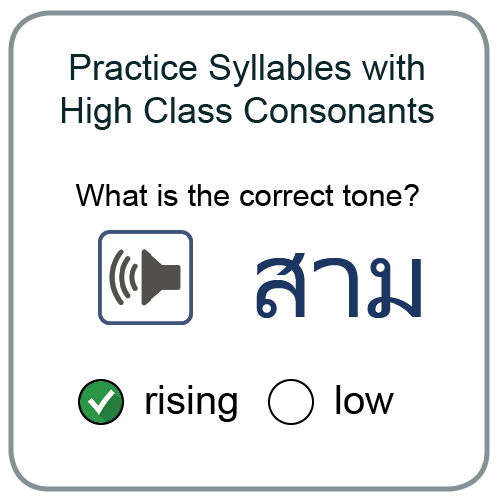In this chapter you will learn to put everything that you have learned about the Thai consonants together with vowels and the final consonant sounds to learn the tone rules, then learn how these rules are modified using one of the four Thai tone marks.

##### Tone Rules for High Class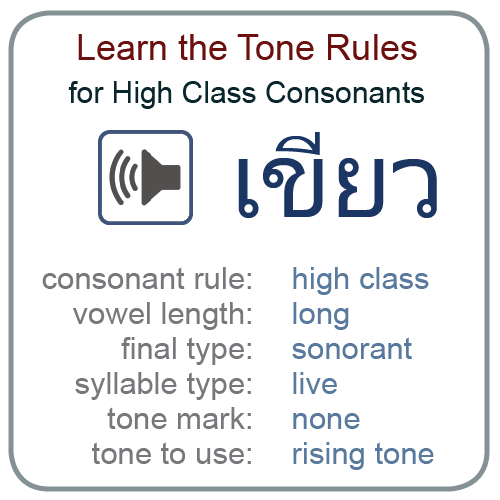In this chapter you will learn to put everything that you have learned about the Thai consonants together with vowels and the final consonant sounds to learn the tone rules, then learn how these rules are modified using one of the four Thai tone marks.

##### Practice Low Class Syllables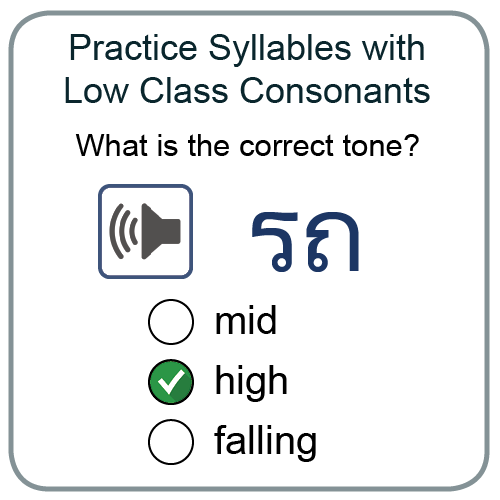In this chapter you will learn to put everything that you have learned about the Thai consonants together with vowels and the final consonant sounds to learn the tone rules, then learn how these rules are modified using one of the four Thai tone marks.

##### Tone Rules for Low Class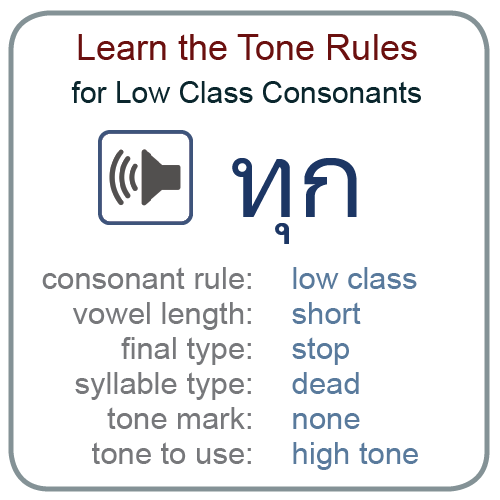In this chapter you will learn to put everything that you have learned about the Thai consonants together with vowels and the final consonant sounds to learn the tone rules, then learn how these rules are modified using one of the four Thai tone marks.

##### Tone Rule Practice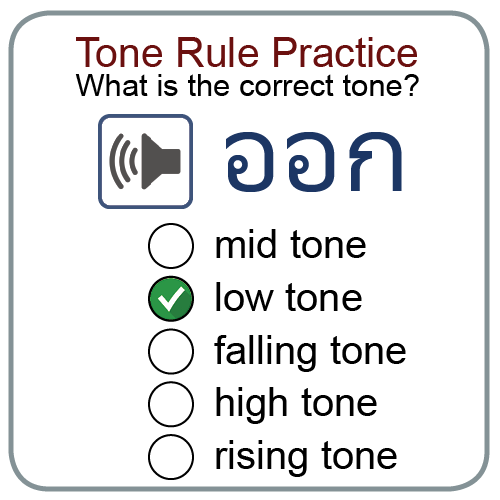In this chapter you will learn to put everything that you have learned about the Thai consonants together with vowels and the final consonant sounds to learn the tone rules, then learn how these rules are modified using one of the four Thai tone marks.

© 2017 - 2019. Thailand Interactive Publishing.

Top